Optimal Power Flow of the Algerian Electrical Network using an Ant Colony Optimization Method

Tarek BOUKTIR and Linda SLIMANI

Department of Electrical Engineering, University of Oum El Bouaghi

Department of Electrical Engineering, University of Setif, Algeria

This paper presents solution of optimal power flow (OPF) problem of a power system via an Ant Colony Optimization Meta-heuristic method. The objective is to minimize the total fuel cost of thermal generating units and also conserve an acceptable system performance in terms of limits on generator real and reactive power outputs, bus voltages, shunt capacitors/reactors, transformers tap-setting and power flow of transmission lines. Simulation results on the Algerian Electrical Network show that the Ant Colony Optimization method converges quickly to the global optimum.

Keywords

Optimal power flow; Power systems; Algerian electrical network; Ant colony optimization

1. Introduction

The optimal power flow has been frequently solved using classical optimization methods. The OPF has been usually considered as the minimization of an objective function representing the generation cost and/or the transmission loss. The constraints involved are the physical laws governing the power generation-transmission systems and the operating limitations of the equipment.

Effective optimal power flow is limited by (i) the high dimensionality of power systems and (ii) the incomplete domain dependent knowledge of power system engineers. The first limitation is addressed by numerical optimization procedures based on successive linearization using the first and the second derivatives of objective functions and their constraints as the search directions or by linear programming solutions to imprecise models [1-4]. The advantages of such methods are in their mathematical underpinnings, but disadvantages exist also in the sensitivity to problem formulation, algorithm selection and usually converge to local minima . The second limitation, incomplete domain knowledge, precludes also the reliable use of expert systems where rule completeness is not possible.

In the evolutionary and adaptive algorithms one of the most recent is the Ant Colony Optimization (ACO) computational paradigm introduced by Marco Dorigo in his Ph.D. thesis in 1992 , and expanded it in his further work, as summarized in [7, 8, 9].

ACO offer a new powerful approach to these optimization problems made possible by the increasing availability of high performance computers at relatively low costs.

As the name suggests, these algorithms have been inspired in the real ant colonies behavior. When searching for food, ants initially explore the area surrounding their nest in a random manner. As soon as an ant finds a food source, it evaluates quantity and quality of the food and carries some of the found food to the nest. During the return trip, the ant deposits a chemical pheromone trail on the ground. The quantity of pheromone deposited, which may depend on the quantity and quality of the food, will guide other ants to the food source. The indirect communication between the ants via the pheromone trails allows them to find shortest paths between their nest and food sources. This functionality of real ant colonies is exploited in artificial ant colonies in order to solve global optimization searching problems when the closed-form optimization technique cannot be applied.

ACO is characterized by the use of a (parameterized) probabilistic model that is used to generate solutions to the problem under consideration. The probabilistic model is called the pheromone model. The pheromone model consists of a set of model parameters, which are called the pheromone trail parameters. The pheromone trail parameters have values, called pheromone values. At run-time, ACO algorithms try to update the pheromone values in such a way that the probability to generate high-quality solutions increases over time. The pheromone values are updated using previously generated solutions. The update aims to concentrate the search in regions of the search space containing high-quality solutions. In particular, the reinforcement of solution components depending on the solution quality is an important ingredient of ACO algorithms. It implicitly assumes that good solutions consist of good solution components. To learn which components contribute to good solutions can help to assemble them into better solutions.

In general, the ACO approach attempts to solve an optimization problem by repeating the following two steps.

1.      Candidate solutions are constructed using a pheromone model; that is, a parameterized probability distribution over the solution space;

2.      The candidate solutions are used to modify the pheromone values in a way that is deemed to bias future sampling toward high-quality solutions.

ACO methods have been successfully applied to diverse combinatorial optimization problems including travelling salesman [10, 11], quadratic assignment [12, 13], vehicle routing [14, 15, 16], telecommunication networks , graph colouring , constraint satisfaction , Hamiltonian graphs , and scheduling [21, 22, 23].

In a previous paper , the authors have proposed the use of a genetic algorithm with real coding on the optimal power flow problem using as objective function the minimization of the fuel cost and NOx emission control. More than 6 small-sized test cases were used to demonstrate the performance of the proposed algorithm. Consistently acceptable results were observed. In other paper  we have presented the results of the application of GA using real coding on the Algerian Electrical Network.

In this paper, we showed the application of the ant colony optimization algorithms in the Optimal Power Flow (OPF) on the Algerian Electrical Network.

The ACO is more likely to converge toward the global solution because it, simultaneously, evaluates many points in the parameter space. It does not need to assume that the search space is differentiable or continuous. To accelerate the processes of ACO-OPF, the controllable variables are decomposed to active constraints that effect directly the cost function are included in the ACO process and passive constraints which are updating using a conventional load flow program, only, one time after the convergence on the ACO-OPF. The slack bus parameter would be recalculated in the load flow process to take the effect of the passive constraints.

The algorithm was developed in an Object Oriented fashion, in the C++ programming language .

2. Problem Formulation

The standard OPF problem can be written in the following form,(1)

where F(x) the objective function, h(x) represents the equality constraints, g(x) represents the inequality constraints and is x is the vector of the control variables, that is those which can be varied by a control center operator (generated active and reactive powers, generation bus voltage magnitudes, transformers taps etc.).

The essence of the optimal power flow problem resides in reducing the objective function and simultaneously satisfying the load flow equations (equality constraints) without violating the inequality constraints

Objective Function

The most commonly used objective in the OPF problem formulation is the minimization of the total cost of real power generation. The individual costs of each generating unit are assumed to be function, only, of active power generation and are represented by quadratic curves of second order. The objective function for the entire power system can then be written as the sum of the quadratic cost model at each generator: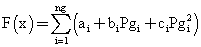(2)

where ng is the number of generation including the slack bus. Pgi is the generated active power at bus i.

ai, bi and ci are the unit costs curve for ith generator.

Types of Equality Constraints

While minimizing the cost function, it is necessary to make sure that the generation still supplies the load demands (Pd) plus losses in transmission lines. Usually the power flow equations are used as equality constraints: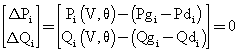(3)

where active and reactive power injection at bus i are defined in the following equation:(4)

where gis is the conductance, bij is the susceptance, Vi is voltage magnitude at the bus i and q ij is the bus voltage phase angle.

Types of Inequality Constraints

The inequality constraints of the OPF reflect the limits on physical devices in the power system as well as the limits created to ensure system security. The most usual types of inequality constraints are upper bus voltage limits at generations and load buses, lower bus voltage limits at load buses, var. limits at generation buses, maximum active power limits corresponding to lower limits at some generators, maximum line loading limits and limits on tap setting of TCULs and phase shifter. The inequality constraints on the problem variables considered include:

• Upper and lower bounds on the active generations at generator buses:

Pgimin£ Pgi £ Pgimax , i = 1, ng                                                                      (5)

• Upper and lower bounds on the reactive power generations at generator buses and reactive power injection at buses with VAR compensation:

Qgimin£ Qgi£ Qgimax , i = 1, npv                                                                    (6)

• Upper and lower bounds on the voltage magnitude at the all buses:

Vimin£ Vi £ Vimax , i = 1, nbus                                                                        (7)

• Upper and lower bounds on the bus voltage phase angles:

θimin£ θi £ θimax , i = 1, nbus                                                                          (8)

• Upper and lower bounds on branch MW/MVAR/MVA flows may come from thermal ratings of conductors, or they may be set to a level due to system stability concerns: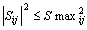(9)

It can be seen that the generalized objective function F is a non-linear, the number of the equality and inequality constraints increase with the size of the power distribution systems. Applications of a conventional optimization technique such as the gradient-based algorithms to a large power distribution system with a very non-linear objective functions and great number of constraints are not good enough to solve this problem. Because it depend on the existence of the first and the second derivatives of the objective function and on the well computing of these derivative in large search space.

3. Ant Colony Optimization in Optimal Power Flow

Description of ant colony optimization method

In the ant colony optimization (ACO), a colony of artificial ants cooperates in finding good solutions to difficult optimization problems. Cooperation is a key design component of ACO algorithms: The choice is to allocate the computational resources to a set of relatively simple agents (artificial ants) that communicate indirectly by stigmergy. Good solutions are an emergent property of the agent’s cooperative interaction.

Artificial ants have a double nature. On the one hand, they are an abstraction of those behavioral traits of real ants which seemed to be at the heart of the shortest path finding behavior observed in real ant colonies. On the other hand, they have been enriched with some capabilities which do not find a natural counterpart. In fact, we want ant colony optimization to be an engineering approach to the design and implementation of software systems for the solution of difficult optimization problems. It is therefore reasonable to give artificial ants some capabilities that, although not corresponding to any capacity of their real ant’s counterparts, make them more effective and efficient.

The objective function to be minimized, as in the traveling salesman problem, is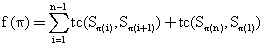(10)

where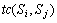is the transition cost between state i and j, and p(i) for i=1,n defines a permutation.

The number of ants is m and given by: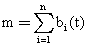(11)

where bi(t) is the number of the ants in state i at time t.

Each ant generates a complete tour by choosing the cities according to a probabilistic state rule. Mathematically, the probability with which ant k in city r chooses to move to the city s is :(12)

where t is the pheromone, h is the visibility which is the inverse of the distance d(r,s), Jk(r) is the set of cities that remain to be visited by ant k positioned on city r , a and b are two coefficients which make the pheromone information or the visibility information more important with respect to one another and the parameter g>0 determines the relative influence of pheromone values corresponding to earlier decisions, i.e., preceding places in the permutation.

A value g=1 results in unweighted summation evaluation, i.e., every tir ,i £r is given the same influence. A value g < 1 (g > 1) gives pheromone values corresponding to earlier decisions less (respectively more) influence.

The best solutions found so far and in the current generation are used to update the pheromone information. However, before that, some portion of pheromone is evaporated according to: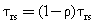(13)

where r is the evaporation rate with 0 £ r < 1 and (1- r) is the trail persistence. The reason for this is that old pheromone should not have too strong an influence on the future.

Let trs(t) be the intensity of trail on edge (r,s) at time t. Each ant at time t chooses the next city, where it will be at time t+1. Therefore, after each cycle, i.e., after each ant has determined a tour, the pheromone trail is updated using the founded solutions according to the following formula: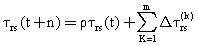(14)

where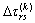is the contribution of the ant k to the pheromone trial between cities r and s.

Usually,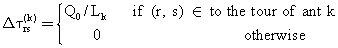(15)

where Q0 is a constant related to the amount of pheromone laid by ants and Lk is the tour length of the the k-th ant.

The process is then iterated and the algorithm runs until some stopping criterion is met, e.g., a certain number of generations have been done or the average quality of the solution found by the ants of a generation has not changed for several generations.

ACO Applied to Optimal Power Flow

Our objective is to minimize the objective function of the OPF defined by (2), using into account the equality constraints (3), and the inequality constraints (5)-(9).

The cost function implemented in ACO is defined as: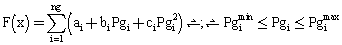(16)

where, only equations (2) and (5) are considered.

The search of the optimal parameters set is performed using into account a part of the equality constraints (3) which present the active power transmission losses (PL) to be deal with in feasible region.

These losses are represented as a penalty vector given by: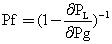(17)

The transmission loss of a power system PL can be calculated by the B-Coefficients method  and given by: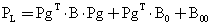(18)

where Pg is a ng-dimensional column vector of the generator power of the units, PgT is the associate matrix of Pg, B is an ng´ng coefficients matrix, B0 is an ng-dimensional coefficient column vector and B00 is a coefficient.

Our objective is to search (Pgi) set in their admissible limits to achieve the optimization problem of OPF. At initialization phase, (Pgi) is selected randomly between Pgimin and Pgimax.

The use of penalty functions in many OPF solutions techniques to handle inequality constraints can lead to convergence problem due to the distortion of the solution surface. In this method only the active power of generators are used in the cost function. And the inequality constraints are scheduled in the load flow process. Because the essence of this idea is that the constraints are partitioned in two types of constraints, active constraints are checked using the ACO-OPF procedure and the reactive constraints are updating using an efficient Newton-Raphson Load flow procedure.

After the search goal is achieved, or an allowable generation is attained by the ACO algorithm. It is required to performing a load flow solution in order to make fine adjustments on the optimum values obtained from the ACO-OPF procedure. This will provide updated voltages, angles and transformer taps and points out generators having exceeded reactive limits. to determining all reactive power of all generators and to determine active power that it should be given by the slack generator using into account the deferent reactive constraints. Examples of reactive constraints are the min and the max reactive rate of the generators buses and the min and max of the voltage levels of all buses. All these require a fast and robust load flow program with best convergence properties. The developed load flow process is based upon the full Newton-Raphson algorithm using the optimal multiplier technique [26, 27].

There are few parameters that to be set for the ant algorithm; these parameters are: r the evaporation rate, m the numbers of ants in the colony, and a and b two coefficients. In the OPF case these values were obtained by a preliminary optimization phase, in which we found that the experimental optimal values of the parameters were largely independent of the problem. By letting the values of a and b change with respect to one another. We observe that the best value for a is 1 and the value of b is 2. The initial pheromone t0 is given by t0 = (ng·L)-1, where L is the tour length produced by the nearest neighbor heuristic. Q0 and r are set to the following values: Q0=0.9 and r=0.1. The number of ants used is m=20. Regarding their initial positioning, ants are placed randomly, with at most one ant in each generator unit.

A local improvement method suggested by Johnson & McGeoch  called the restricted 3-opt method has been adapted for use in the ACO. It involves successive arc-exchanges in an attempt to improve a candidate solution. But we choose a limited number of exchanges in order to avoid over-long computation times. The local search is applied once the solution is built and the results of this phase are used to update the pheromone trails.

4. Application Study

The ACO-OPF is coded in Borland C++ Builder version 5, and run using an Intel Pentium 4, 1500 MHz PC with 128 MB RAM. All computations use real float point precision without rounding or truncating values. More than 6 small-sized test cases were used to demonstrate the performance of the proposed algorithm. Consistently acceptable results were observed.

The ACO-OPF has been tested on a part of the Algerian network (figure 1). It consists of 59 buses, 83 branches (lines and transformers) and 10 generators.

The table 1 shows the technical and economic parameters of the ten generators of the Algerian electrical network. Knowing that the generator of the bus of № = 13 is not in service.

Table 1. Generators parameters of the Algerian Electrical Network

 Bus Number Pmin [MW] Pmax [MW] a [\$/hr] b [\$/MWhr] c [\$/MW2hr] 1 8 72 0 1.50 0.0085 2 10 70 0 2.50 0.0170 3 30 510 0 1.50 0.0085 4 20 400 0 1.50 0.0085 13 15 150 0 2.50 0.0170 27 10 100 0 2.50 0.0170 37 10 100 0 2.00 0.0030 41 15 140 0 2.00 0.0030 42 18 175 0 2.00 0.0030 53 30 450 0 1.50 0.0085

The comparisons of the results obtained by the application of OPF via the ACO with those found by the GA of real type are reported in the table 2. The results obtained with the ACO are better than those obtained by the GA.

The ACO gives a more important profit in fuel cost of 121.4 \$/h (6.27%). The optimum value 1815.7 \$/h has been obtained after 25 seconds. This value take into account the exact cost of the total real power losses by proceeding to a power flow calculation of type Newton-Raphson in order to compute the reactive generated powers, the voltages of all buses and readjust slack generator that takes in consideration the exact losses of real powers. The convergence of the method of N-R is achieved after 4 iterations and 0.01 sec.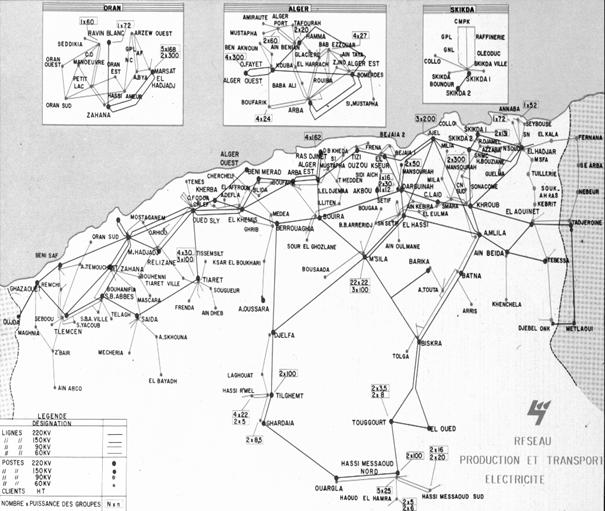Figure 1. Topology of the Algerian production and transmission network before 1997

Table 2. Comparison of the results obtained by the ACO and GA methods on a part of the Algerian electrical network

 Genetic method ACO method Pg 1 70.573 64.01 Pg 2 56.57 22.75 Pg 3 89.27 82.37 Pg 4 78.22 46.21 Pg 13 0.00 0.00 Pg 27 57.93 47.05 Pg 37 39.55 65.56 Pg 41 46.40 39.55 Pg 42 63.58 154.23 Pg 53 211.58 202.36 PD (MW) 684.10 684.1 PL (MW) 29.58 39.98 Cost(\$/h) 1937.10 1815.7

Conclusions

An ant Colony Optimization approach to the Optimal Power Flow problem is introduced and tested. As a study case, the Algerian Electrical Network with 59 buses, 83 branches (lines and transformers) and 10 generators has been selected. The simulation results show that for medium-scale system an ACO algorithm code can give a best result with reduced time. It is recommended to indicate that in large-scale system the number of constraints is very large consequently the ACO accomplished in a large CPU time. To save an important CPU time, the constraints are to be decomposing in active constraints and reactive ones. The active constraints are the parameters whose enter directly in the cost function and the reactive constraints are infecting the cost function indirectly. With this approach, only the active constraints are taken to calculate the optimal solution set. And the reactive constraints are taking in an efficient load flow by recalculate active power of the slack bus.

References

          Dommel H. W., Tinney W. F., Optimal Power Flow Solutions, IEEE Transactions on power apparatus and systems, vol. PAS.87, No. 10, 1968, p. 1866-1876.

          Lee K. Y., Park Y. M., and Ortiz J. L., A United Approach to Optimal Real and Reactive Power Dispatch, IEEE Transactions on Power Systems, Vol. PAS-104, 1985, p. 1147-1153.

          Sasson M., Non linear Programming Solutions for load flow, minimum loss, and economic dispatching problems, IEEE Trans. on Power Apparatus and Systems, vol. Pas-88, No. 4, 1969.

          Bouktir T., Belkacemi M., Zehar K., Optimal power flow using modified gradient method, Proceedings ICEL’2000, U.S.T. Oran, Algeria, 2000, p. 436-442.

          Fletcher R., Practical Methods of Optimisation, John Willey & Sons, 1986.

          Dorigo M., Optimization, learning, and natural algorithms, Ph.D. Dissertation (in Italian), Dipartimento di Elettronica, Politecnico di Milano, Italy, 1992.

          Dorigo M. and Di Caro G., The ant colony optimization metaheuristic, in Corne D., Dorigo M., and Glover F., New Ideas in Optimization, McGraw-Hill, 19997, p. 11-32.

          Dorigo M., Di Caro G., and Gambardella L. M., Ant algorithms for discrete optimization, Artificial Life, Vol. 5, No. 2, 1999, p. 137-172.

          Dorigo M., Maniezzo V., and Colorni A., Ant system: optimization by a colony of cooperating agents, IEEE Trans. System Man. and Cybernetics, Part B: Cybernetics, Vol. 26, No. 1, 1996, p. 29-41.

      Dorigo M. and Gambardella L. M., Ant colonies for the traveling salesman problem, BioSystems, Vol. 43, 1997, p. 73-81.

      Dorigo M. and Gambardella L. M., Ant colony system: a cooperative learning approach to the traveling salesman problem, IEEE Transactions on Evolutionary Computation, Vol. 1, No. 1, 1997, p. 53-66.

      Maniezzo V. and Colorni A., The ant system applied to the quadratic assignment problem, IEEE Trans. Knowledge and Data Engineering, Vol. 11, No. 5, 1999, p. 769-778.

      Stuetzle T. and Dorigo M., ACO algorithms for the quadratic assignment problem, in Corne D., Dorigo M., and Glover F., New Ideas in Optimization, McGraw-Hill, 1999.

      Bullnheimer B., Hartl R. F., and Strauss C., Applying the ant system to the vehicle routing problem, in Voss S., Martello S., Osman I. H., and Roucairol C., Meta-Heuristics: Advances and Trend in Local Search Paradigms for Optimization, Kluwer, 1999, p. 285-296.

      Bullnheimer B., Hartl R. F., and Strauss C., An improved ant system algorithm for the vehicle routing problem, Ann. Oper. Res., Vol. 89, 1999, p. 319-328.

      Gambardella L. M., Taillard E., and Agazzi G., MACS-VRPTW a multiple ant colony system for vehicle routing problems with time windows, in Corne D., Dorigo M., and Glover F., New Ideas in Optimization, McGraw-Hill, 1999, p. 63-76.

      Di Caro G. and Dorigo M., Ant colonies for adaptive routing in packet-switched communication networks, Proc. 5th Int. Conf. Parallel Problem Solving From Nature, Amsterdam, The Netherlands, 1998, p. 673-682.

      Costa D. and Hertz A., Ants can color graphs, J. Oper. Res. Soc., Vol. 48, 1997, p. 295-305.

      Schoofs L. and Naudts B., Ant colonies are good at solving constraint satisfaction problems, Proc. 2000 Congress on Evolutionary Computation, San Diego, CA, 2000, p. 1190-1195.

       Wagner I. A. and Bruckstein A. M., Hamiltonian(t)-an ant inspired heuristic for recognizing Hamiltonian graphs, Proc. 1999 Congress on Evolutionary Computation, Washington, D.C., 1999, p. 1465-1469.

      Bauer A., Bullnheimer B., Hartl R. F., and Strauss C., Minimizing total tardiness on a single machine using ant colony optimization, Central Eur. J. Oper. Res., Vol. 8, No. 2, 2000, p. 125-141.

      Colorni A., Dorigo M., Maniezzo V., and Trubian M., Ant system for job-shop scheduling, Belgian J. Oper. Res., Statist. Comp. Sci. (JORBEL), Vol. 34, No. 1, 1994, p. 39-53.

       Den Besteb M., Stützle T., and Dorigo M., Ant colony optimization for the total weighted tardiness problem, Proc. 6th Int. Conf. Parallel Problem Solving from Nature, Berlin, 2000, p. 611-620.

      Merkle D. and Middendorf M., An ant algorithm with a new pheromone evaluation rule for total tardiness problems, Proceedings of the EvoWorkshops 2000, Berlin, Germany: Springer-Verlag, Vol. 1803 then Lecture Notes in Computer Science, 2000, p. 287-296.

      Del Toro V., Electric Power Systems, Vol. 2, Prentice Hall, Englewood Cliffs, New Jersey, USA, 1992.

      Stagg G. W., El Abiad A. H., Computer methods in power systems analysis, McGraw Hill International Book Company, 1968.

      Kumar S., Billinton R., Low bus voltage and ill-conditioned network situation in a composite system adequacy evaluation, IEEE Transactions on Power Systems, Vol. PWRS-2, No. 3, 1987.

      Johnson D.S. & McGeoch L.A., The traveling salesman problem: a case study in local optimization, in Aarts E. H. L. and Lenstra J. K., Local Search in Combinatorial Optimization, John Wiley and Sons, p. 215-310, 1997.

      Terra L., Short M., Security constrained reactive power dispatch, IEEE Transaction on Power Systems, Vol. 6, No. 1, p. 109-117, 1991.

      Bouktir T. and Belkacemi M., Object-Oriented Optimal Power Flow, Electric Power Components and Systems, vol. 31, no. 6, p. 525-534, 2003.

      Yuryevich J., Wong K. P., Evolutionary Programming Based Optimal Power Flow Algorithm, IEEE Transaction on Power Systems, vol. 14, no. 4, p. 1245-1250, 1999.

      Lai L.L., Ma J. T., Yokoma R., Zhao M., Improved genetic algorithms for optimal power flow under both normal and contingent operation states, Electrical Power & Energy System, Vol. 19, No. 5, p. 287-292, 1997.

      Bouktir T. and Slimani L., Economic Power Dispatch of Power Systems with a NOx emission Control via an Evolutionary Algorithm, WSEAS TRANSACTIONS on SYSTEMS, Issue 2, Vol. 3, p. 849-854, 2004.

      Bouktir T. and Slimani L., Optimal power flow of the Algerian Electrical Network Using Genetic Algorithms, WSEAS TRANSACTIONS on CIRCUIT and SYSTEMS, Issue 6, Vol. 3, p. 1478-1482, 2004.